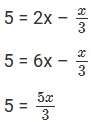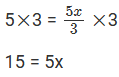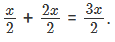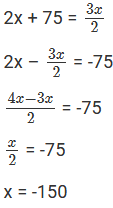×#### Thank you for registering.

One of our academic counsellors will contact you within 1 working day.

Click to Chat

1800-1023-196

+91-120-4616500

CART 0

• 0

MY CART (5)

Use Coupon: CART20 and get 20% off on all online Study Material

ITEM
DETAILS
MRP
DISCOUNT
FINAL PRICE
Total Price: Rs.

There are no items in this cart.
Continue Shopping• Complete JEE Main/Advanced Course and Test Series
• OFFERED PRICE: Rs. 15,900
• View Details

```Chapter 8: Linear Equations in One Variable Exercise – 8.4

Question: 1

If 5 is subtracted from three times a number, the result is 16. Find the number.

Solution:

Let the required number be ‘x’. Then, 5 subtracted from 3 times x = 3x – 5.

=> 3x – 5 = 16

Adding 5 to both sides, we get = 3x – 5 + 5 = 16 + 5

=> 3x = 21

Dividing both sides by 3, we get

3x/3 = 21/3

x = 7

Thus, the required number is 7.

Question: 2

Find the number which when multiplied by 7 is increased by 78.

Solution:

Let the required number be ‘x’. Thus, when multiplied by 7, it gives 7x, and x increases by 78.

7x = x + 78

Transposing x to LHS, we get

7x – x = 78

6x = 78

Dividing both sides by 6, we get

6x/6 = 78/6

x =13

Thus, the required number is 13.

Question: 3

Find three consecutive natural numbers such that the sum of the first and second is 15 more than the third.

Solution:

Let the first number be ‘x’. Hence, the second number = x + 1 and the third number = x + 2.

=> Sum of first and second numbers = (x) + (x + 1).

According to question:

(x) + (x + 1) = 15 + (x + 2)

=> 2x + 1 = 17 + x

Transposing x to LHS and 1 to RHS, we get

=> 2x – x = 17 – 1

=> x = 16

So, first number = x = 16, second number = x + 1 = 16 + 1 = 17 and third number = x + 2 = 16 + 2 = 18

Thus, the required consecutive natural numbers are 16, 17 and 18.

Question: 4

The difference between two numbers is 7. Six times the smaller plus the larger is 77. Find the numbers.

Solution:

Let the smaller number be ‘x’. So, the larger number = x + 7.

According to question:

6x + (x + 7) = 77

6x + x + 7 = 77

7x + 7 = 77

Subtracting 7 from both sides, we get

7x + 7 – 7 = 77 – 7

7x = 70

Dividing both sides by 7, we get

7x/7 = 70/7

x = 10

Thus, the smaller number = x = 10, and the larger number = x + 7 = 10 + 7 = 17.

The two required numbers are 10 and 17.

Question: 5

A man says, “I am thinking of a number. When I divide it by 3 and then add 5, my answer is twice the number I thought of ”. Find the number.

Solution:

Let the number thought of by the man be ‘x’.

So, According to question:

x/3 + 5 = 2x

Transposing x/3 to RHS, we getMultiplying both sides by 3, we getDividing both sides by 5, we get

15/5 = 5x/5

x = 3

Thus, the number thought of by the man is 3.

Question: 6

If a number is tripled and the result is increased by 5, we get 50. Find the number.

Solution:

Let the required number be ‘x’.

So, According to question:

=> 3x + 5 = 50

Subtracting 5 from both sides, we get

=> 3x + 5 – 5 = 50 – 5

=> 3x = 45

Dividing both sides by 3, we get

3x/3 = 45/3

x = 15

Thus, the required number is 15.

Question: 7

Shikha is 3 years younger to her brother Ravish. If the sum of their ages is 37 years, what are their present ages?

Solution:

Let the present age of Shikha = ‘x’ years.

So, the present age of Shikha’s brother Ravish = (x + 3) years.

So, sum of their ages = x + (x+ 3)

=> x +(x + 3) = 37

=> 2x + 3 = 37

Subtracting 3 from both sides, we get

=> 2x+ 3 – 3 = 37 – 3

=> 2x = 34

Dividing both sides by 2, we get

=> 2x/2 = 34/2

=> x = 17

So, the present age of Shikha = 17 years, and the present age of Ravish = x + 3 = 17 + 3 = 20 years.

Question: 8

Mrs. Jain is 27 years older than her daughter Nilu. After 8 years she will be twice as old as Nilu. Find their present ages.

Solution:

Let the present age of Nilu = ‘x’ years.

Therefore, the present age of Nilu’s mother, Mrs. Jain = (x + 27) years.

So, after 8 years,

Nilu’s age = (x + 8), and Mrs. Jain’s age = (x + 27 + 8) = (x + 35) years

=> x + 35 = 2(x + 8)

Expanding the brackets, we get

=> x + 35 = 2x + 16

Transposing x to RHS and 16 to LHS, we get

=> 35 – 16 = 2x – x

=> x = 19

So, the present age of Nilu = x = 19 years, and the present age of Nilu’s mother = x+ 27 = 19 + 27 = 46 years.

Question: 9

A man is 4 times as old as his son. After 16 years, he will be only twice as old as his son. Find the their present ages.

Solution:

Let the present age of the son = ‘x’ years.

Therefore, the present age of his father = ‘4x’ years.

So, after 16 years,

Son’s age = (x + 16) and father’s age = (4x + 16) years

According to question:

=> 4x + 16 = 2(x + 16)

=> 4x + 16 = 2x + 32

Transposing 2x to LHS and 16 to RHS, we get

=> 4x – 2x = 32 – 16

=> 2x = 16

Dividing both sides by 2, we get

2x/2 = 16/2

=> x = 8

So, the present age of the son = x = 8 years, and the present age of the father = 4x = 4(8) = 32 years.

Question: 10

The difference in age between a girl and her younger sister is 4 years. The younger sister in turn is 4 years older than her brother. The sum of the ages of the younger sister and her brother is 16. How old are the three children?

Solution:

Let the age of the girl = ‘x’ years.

So, the age of her younger sister = (x – 4) years.

Thus, the age of the brother = (x – 4 – 4) years = (x – 8) years.

According to question:

(x – 4) + (x – 8) = 16

x + x – 4 – 8 = 16

2x – 12 = 16

Adding 12 to both sides, we get

2x – 12 + 12 = 16 + 12

2x = 28

Dividing both sides by 2, we get

2x/2 = 28/2

x = 14

Thus, the age of the girl = x = 14 years, the age of the younger sister = x – 4 = 14 – 4 = 10 years, and the age of the younger brother = x – 8 = 14 – 8 = 6 years.

Question: 11

One day, during their vacation at a beach resort, Shelia found twice as many sea shells as Anita and Anita found 5 shells more than sandy. Together sandy and Sheila found 16 sea shells. How many did each of them find?

Solution:

Let the number of sea shells found by Sandy = ‘x’.

So, the number of sea shells found by Anita = (x + 5).

The number of sea shells found by Shelia = 2 (x + 5).

According to the question,

=> x + 2(x + 5) = 16

=> x + 2x +10 = 16 = 3x + 10 = 16

Subtracting 10 from both sides, we get

=> 3x + 10 – 10 = 16 – 10

=> 3x = 6

Dividing both sides by 3, we get

=> 3x/6 = 6/3

=> x = 4

Thus, the number of sea shells found by Sandy = x = 4, the number of sea shells found by Anita = x + 5 = 4 + 5 = 9,

and the number of sea shells found by Shelia = 2(x + 5) = 2(4 + 5) = 2(9 ) = 18.

Question: 12

Andy has twice as many marbles as Pandy, and Sandy has half as many has Andy and Pandy put together. If Andy has 75 marbles more than Sandy. How many does each of them have?

Solution:

Let the number of marbles with Pandy = ‘x’.

So, the number of marbles with Andy = ‘2x’.

Thus, the number of marbles with Sandy =According to the question,x = -150

Since, no. of marbles cannot be negative.

Therefore, x = 150

So, Pandy has 150 marbles, Andy has 2x = 2(150) = 300 marbles, and Sandy has 3x/2 = 225 marbles.

Question: 13

A bag contains 25 paise and 50 paise coins whose total value is Rs 30. If the number of 25 paise coins is four times that of 50 paise coins, find the number of each type of coins.

Solution:

Let the number of 50 paise coins = ‘x’.

So, the money value contribution of 50 paise coins = 0.5x.

The number of 25 paise coins = ‘4x’.

The money value contribution of 25 paise coins = 0.25(4x) = x.

According to the question,

0.5x + x = 30

=> 1.5x = 30

Dividing both sides by 1.5, we get

=> 1.5x/1.5= 30/1.5

=> x = 20

Thus, the number of 50 paise coins = ‘x’ = 20, and the number of 25 paise coins = ‘4x’ = 4 (20) = 80.

Question: 14

The length of a rectangular field is twice its breadth. If the perimeter of the field is 228 metres, find the dimensions of the field.

Solution:

Let the breadth of the rectangle = ‘x’ metres.

According to the question,

Length of the rectangle = ‘2x’ metres

Perimeter of a rectangle = 2 (length + breadth)

So, 2 (2x + x) = 228

=> 2 (3x) = 228

=> 6x = 228

Dividing both sides by 6, we get

=> 6x/6 = 228/6

=> x = 38

So, the breadth of the rectangle = x = 38 metres,

and the length of the rectangle = 2x = 2(38) = 76 metres.

Question: 15

There are only 25 paise coins in a purse. The value of money in the purse is Rs 17.50. Find the number of coins in the purse.

Solution:

Let the number of 25-paise coins in the purse be ‘x’.

So, the value of money in the purse = 0.25x.

But 0.25x = 17.5.

Dividing both sides by 0.25, we get

=> 0.25x/0.25 = 17.5/0.25

=> x = 70

Thus, the number of 25-paise coins in the purse = 70.

Question: 16

In a hostel mess, 50 kg rice are consumed everyday. If each student gets 400 gm of rice per day, find the number of students who take meals in the hostel mess.

Solution:

Let the number of students in the hostel be ‘x’.

Quantity of rice consumed by each student = 400 gm.

So, daily rice consumption in the hostel mess = 400(x).

But, daily rice consumption = 50 kg = 50 × 1000 = 50000 gm [since 1 kg = 1000 gm].

According to the question,

400x = 50000

Dividing both sides by 400, we get

=> 400x/400 = 50000/400

=> x = 125

Thus, 125 students have their meals in the hostel mess.

```### Course Features

• 728 Video Lectures
• Revision Notes
• Previous Year Papers
• Mind Map
• Study Planner
• NCERT Solutions
• Discussion Forum
• Test paper with Video Solution